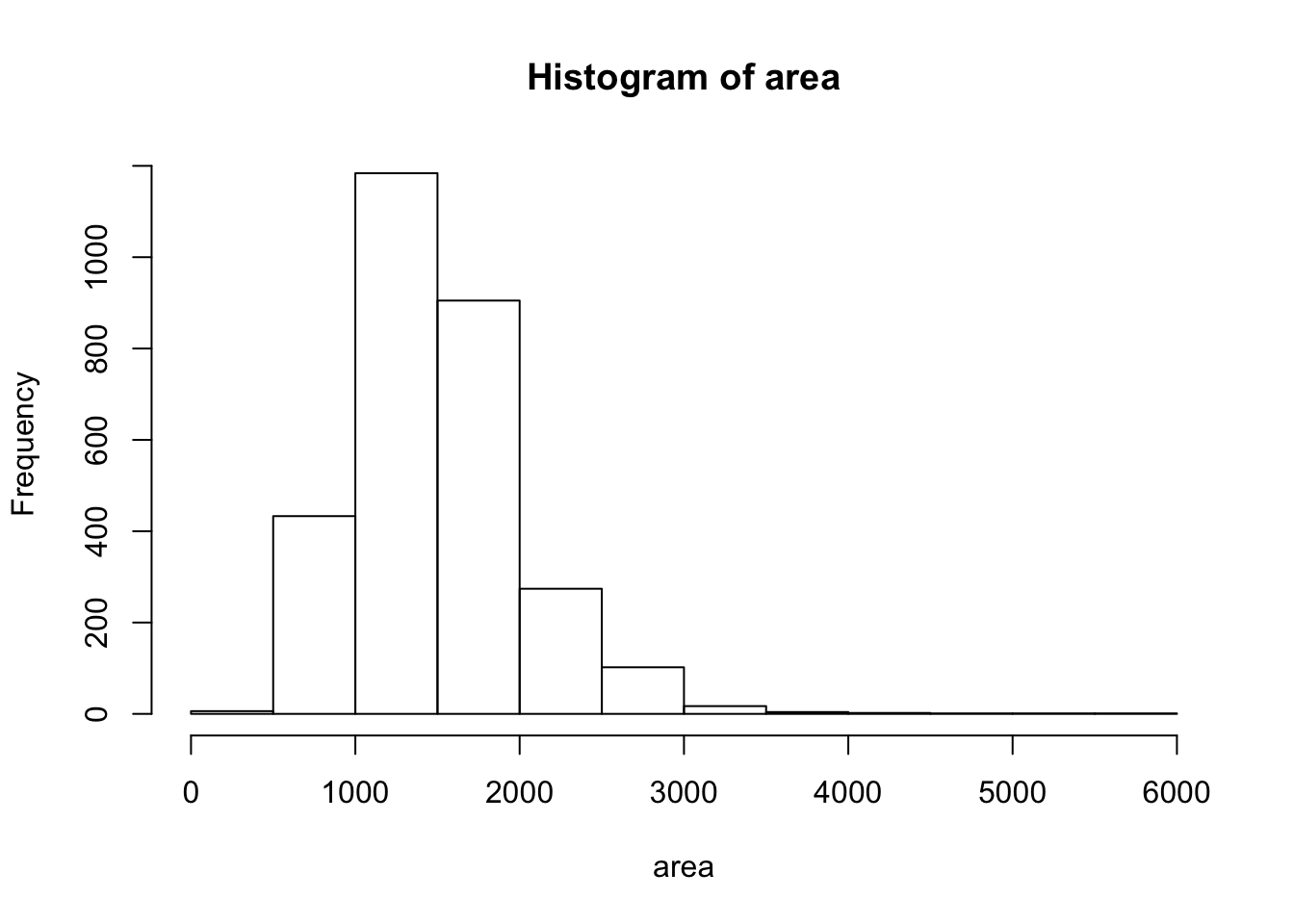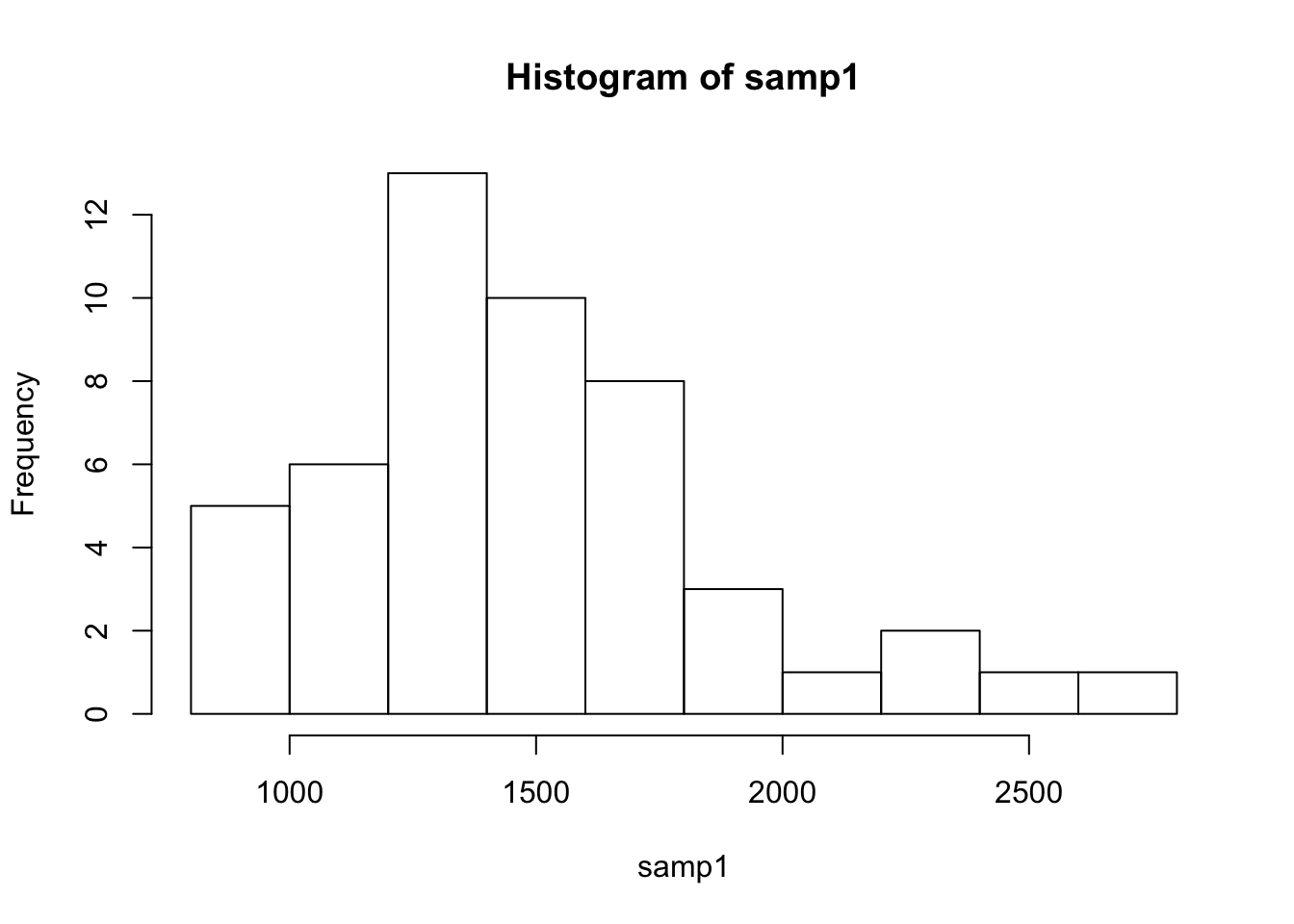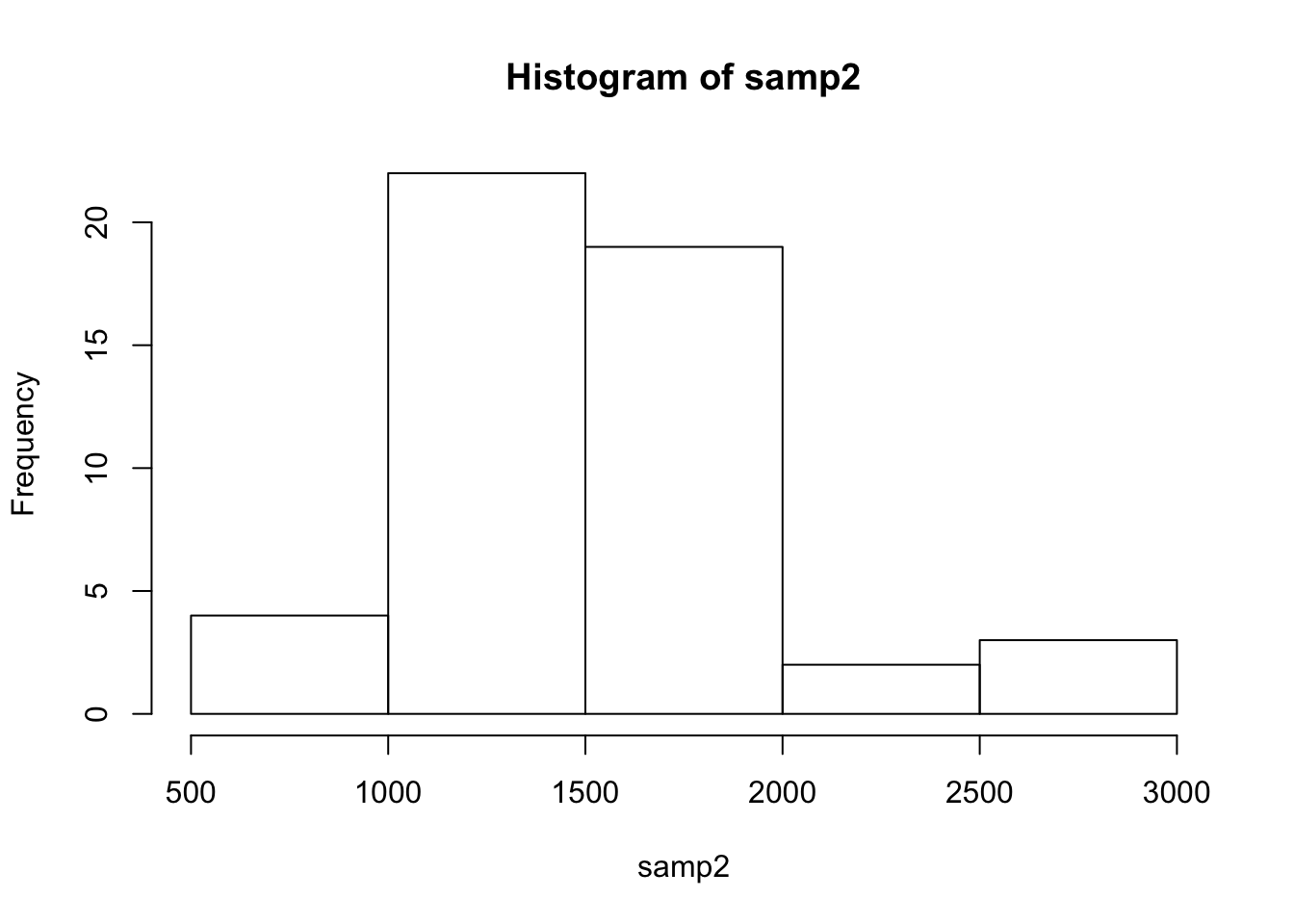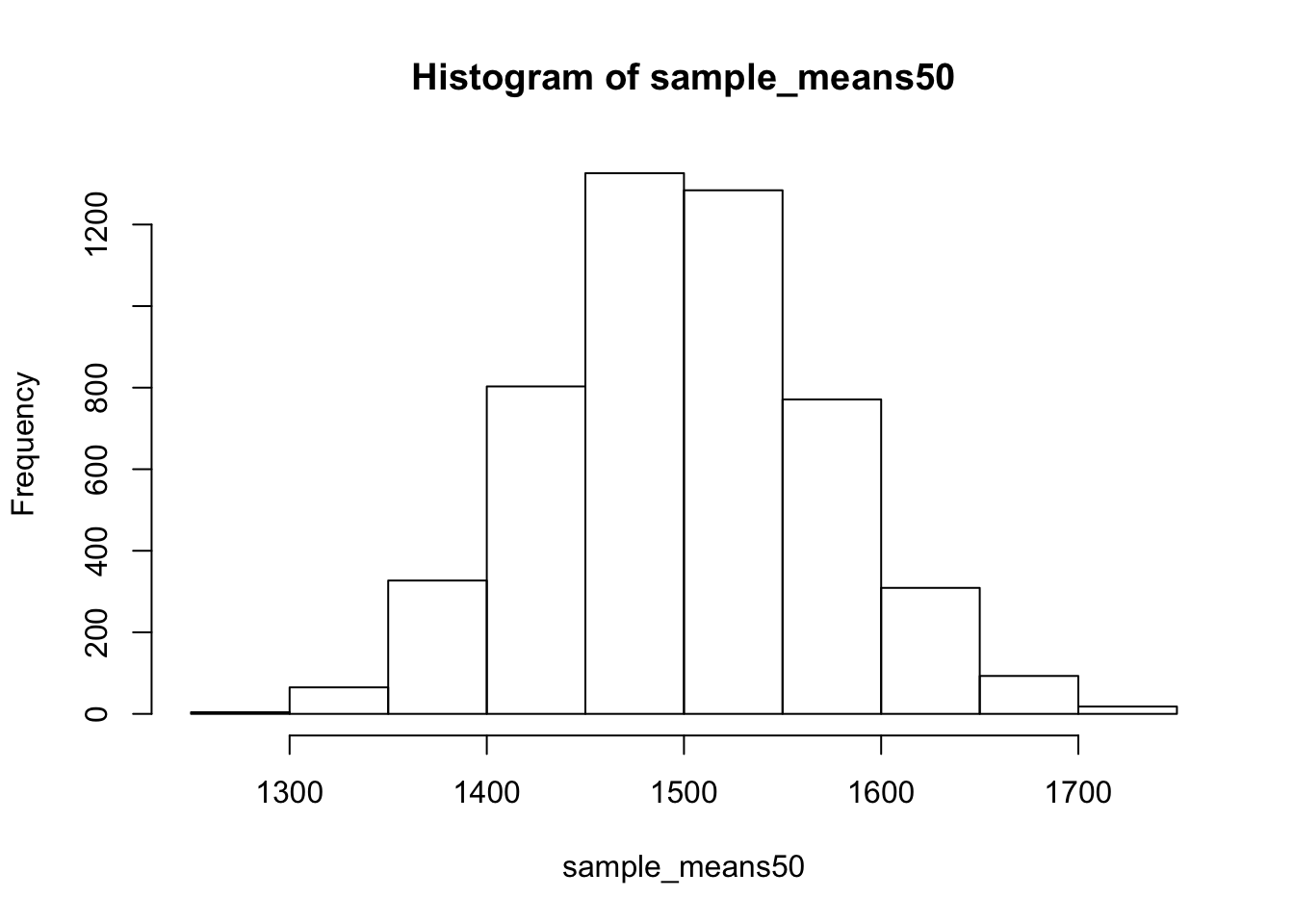In this lab, we investigate the ways in which the statistics from a random sample of data can serve as point estimates for population parameters. We’re interested in formulating a sampling distribution of our estimate in order to learn about the properties of the estimate, such as its distribution.

## The data

We consider real estate data from the city of Ames, Iowa. The details of every real estate transaction in Ames is recorded by the City Assessor’s office. Our particular focus for this lab will be all residential home sales in Ames between 2006 and 2010. This collection represents our population of interest. In this lab we would like to learn about these home sales by taking smaller samples from the full population. Let’s load the data.

``load("more/ames.RData")``

We see that there are quite a few variables in the data set, enough to do a very in-depth analysis. For this lab, we’ll restrict our attention to just two of the variables: the above ground living area of the house in square feet (`Gr.Liv.Area`) and the sale price (`SalePrice`). To save some effort throughout the lab, create two variables with short names that represent these two variables.

``````area <- ames\$Gr.Liv.Area
price <- ames\$SalePrice``````

Let’s look at the distribution of area in our population of home sales by calculating a few summary statistics and making a histogram.

``summary(area)``
``````##    Min. 1st Qu.  Median    Mean 3rd Qu.    Max.
##     334    1126    1442    1500    1743    5642``````
``hist(area)``1. Describe this population distribution.

Question 1 This population distribution seems to be skewed to the right, since the mean is larger than the median and values in the histogram trail to the right.

## The unknown sampling distribution

In this lab we have access to the entire population, but this is rarely the case in real life. Gathering information on an entire population is often extremely costly or impossible. Because of this, we often take a sample of the population and use that to understand the properties of the population.

If we were interested in estimating the mean living area in Ames based on a sample, we can use the following command to survey the population.

``set.seed(1); samp1 <- sample(area, 50)``

This command collects a simple random sample of size 50 from the vector `area`, which is assigned to `samp1`. This is like going into the City Assessor’s database and pulling up the files on 50 random home sales. Working with these 50 files would be considerably simpler than working with all 2930 home sales.

1. Describe the distribution of this sample. How does it compare to the distribution of the population?

Question 2 The distribution of this sample is also skewed to the right. Its mean is larger than the median and many of its values are spread out to the right of the distribution.

``summary(samp1)``
``````##    Min. 1st Qu.  Median    Mean 3rd Qu.    Max.
##     864    1218    1463    1491    1679    2730``````
``hist(samp1)``If we’re interested in estimating the average living area in homes in Ames using the sample, our best single guess is the sample mean.

``mean(samp1)``
``##  1491.38``

Depending on which 50 homes you selected, your estimate could be a bit above or a bit below the true population mean of 1499.69 square feet. In general, though, the sample mean turns out to be a pretty good estimate of the average living area, and we were able to get it by sampling less than 3% of the population.

1. Take a second sample, also of size 50, and call it `samp2`. How does the mean of `samp2` compare with the mean of `samp1`? Suppose we took two more samples, one of size 100 and one of size 1000. Which would you think would provide a more accurate estimate of the population mean?

Question 3 The mean of samp2 is slightly larger than the mean of samp1. The sample of size 1000 would provide a more accurate estimate of the population mean (law of large numbers).

``````set.seed(2); samp2 <- sample(area, 50)
summary(samp2)``````
``````##    Min. 1st Qu.  Median    Mean 3rd Qu.    Max.
##     845    1180    1468    1519    1786    2822``````
``hist(samp2)``Not surprisingly, every time we take another random sample, we get a different sample mean. It’s useful to get a sense of just how much variability we should expect when estimating the population mean this way. The distribution of sample means, called the sampling distribution, can help us understand this variability. In this lab, because we have access to the population, we can build up the sampling distribution for the sample mean by repeating the above steps many times. Here we will generate 5000 samples and compute the sample mean of each.

``````sample_means50 <- rep(NA, 5000)

set.seed(3); for(i in 1:5000){
samp <- sample(area, 50)
sample_means50[i] <- mean(samp)
}

hist(sample_means50)``````If you would like to adjust the bin width of your histogram to show a little more detail, you can do so by changing the `breaks` argument.

``hist(sample_means50, breaks = 25)``